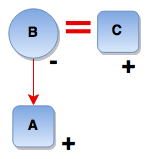# Blood Relations and Family Tree - Logical Reasoning (MCQ) Questions for Q. 29956

1. J + K means J is the son of K
2. J - K means J is the wife of K
3. J x K means J is the brother of K
4. J ÷ K means J is the mother of K
5. J = K means J is the sister of K

What does A + B – C mean?

- Published on 08 May 17

a. C is the father of A
b. C is the uncle of A
c. C is the son of A
d. C is the brother of A

ANSWER: C is the father of A

A + B – C means A is the son of B who is the wife of C i.e. C is the father of A.## ➨ Post your comment / Share knowledgeEnter the code shown above:

(Note: If you cannot read the numbers in the above image, reload the page to generate a new one.)